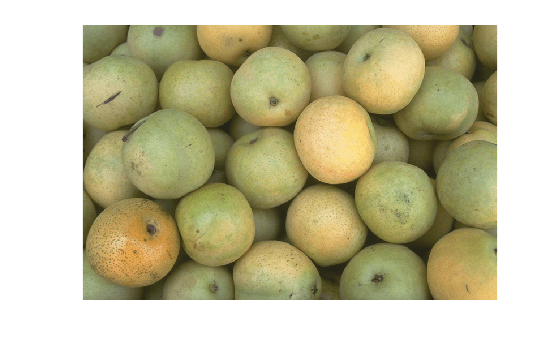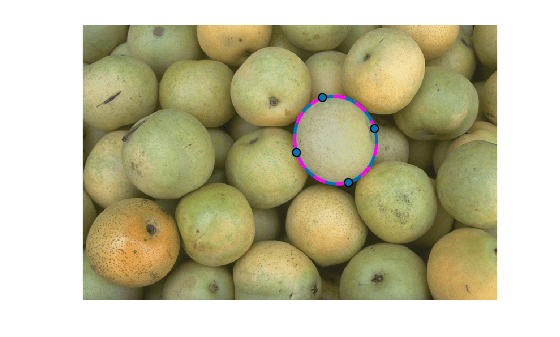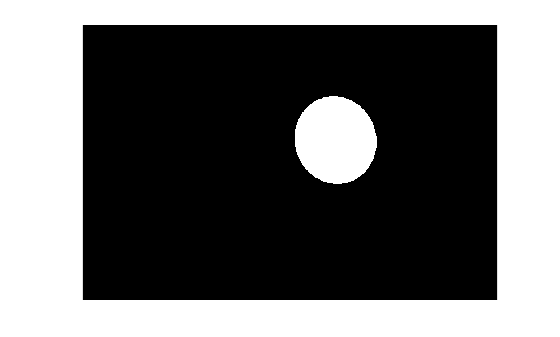## 语法

``bw = createMask(ROI)``
``bw = createMask(ROI,m,n)``
``bw = createMask(ROI,I)``
``bw = createMask(ROI,hImage)``

## 说明

` `bw = createMask(ROI)` 返回二值掩膜图像，其中 ROI 内的像素设置为 `true`，ROI 外的像素设置为 `false`。 `
` `bw = createMask(ROI,m,n)` 返回大小为 `[m,n]` 的二值掩膜图像。`
` `bw = createMask(ROI,I)` 返回二值掩膜图像，其大小与图像 `I` 相同。`
` `bw = createMask(ROI,hImage)` 返回二值掩膜图像，其大小与 `Image` 对象 `hImage` 相同。`

## 示例

```I = imread('pears.png'); imshow(I)``````h = drawellipse('Center',[447 204],'SemiAxes',[78 72], ... 'RotationAngle',287,'StripeColor','m');``````mask = createMask(h); imshow(mask)```## 提示

• 有关对 ROI 边界上的像素进行分类的详细信息，请参阅Classify Pixels That Are Partially Enclosed by ROI

• 如果您指定的掩膜大小与 `ROI` 对象所关联的图像大小不匹配，则 `createMask` 会将掩膜裁剪或零填充到指定的大小。与 ROI 对象相关联的图像为 `ROI.Parent.Children(2,1).CData`